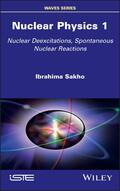John Wiley & Sons Nuclear Physics 1This book presents the foundations of nuclear physics, covering several themes that range from subat.. Product #: 978-1-78630-641-8 Regular price: \$142.06 \$142.06 In Stock

# Nuclear Physics 1

## Nuclear Deexcitations, Spontaneous Nuclear Reactions1. Edition January 2022
356 Pages, Hardcover
Wiley & Sons Ltd

ISBN: 978-1-78630-641-8
John Wiley & Sons

### Price: 152,00 €

Price incl. VAT, excl. Shipping

This book presents the foundations of nuclear physics, covering several themes that range from subatomic particles to stars. Also described in this book are experimental facts relating to the discovery of the electron, positron, proton, neutron and neutrino. The general properties of nuclei and the various nuclear de-excitation processes based on the nucleon layer model are studied in greater depth.

This book addresses the conservation laws of angular momentum and parity, the multipolar transition probabilities E and M, gamma de-excitation, internal conversion and nucleon emission de-excitation processes. The fundamental properties of alpha and ß disintegrations, electron capture, radioactive filiations, and Bateman equations are also examined. Nuclear Physics 1 is intended for high school physics teachers, students, research teachers and science historians specializing in nuclear physics.

Preface ix

Chapter 1. Overview of the Nucleus 1

1.1. Discovery of the electron 2

1.1.1. Hittorf and Crookes experiments 2

1.1.2. Perrin and Thomson experiments 4

1.1.3. Millikan experiment 8

1.2. The birth of the nucleus 12

1.2.1. Perrin and Thomson atomic model 12

1.2.2. Geiger and Marsden experiment 13

1.2.3. Rutherford scattering: Planetary atomic model 14

1.2.4. Rutherford's differential effective cross-section 16

1.3. Composition of the nucleus 22

1.3.1. Discovery of the proton 22

1.3.2. Discovery of the neutron 24

1.3.3. Internal structure of nucleons: u and d quarks 28

1.3.4. Isospin 30

1.3.5. Nuclear spin 31

1.3.6. Nuclear magnetic moment 31

1.4. Nucleus dimensions 33

1.4.2. Nuclear density, skin thickness 35

1.5. Nomenclature of nuclides 39

1.5.1. Isotopes, isobars, isotones 39

1.5.2. Mirror nuclei, Magic nuclei 43

1.6. Nucleus stability 43

1.6.1. Atomic mass unit 43

1.6.2. Segrè diagram, nuclear energy surface 45

1.6.3. Mass defect, binding energy 46

1.6.4. Binding energy per nucleon, Aston curve 49

1.6.5. Separation energy of a nucleon 52

1.6.6. Nuclear forces 54

1.7. Exercises 54

1.8. Solutions to exercises 59

Chapter 2. Nuclear Deexcitations 69

2.1. Nuclear shell model 71

2.1.1. Overview of nuclear models 71

2.1.2. Individual state of a nucleon 72

2.1.3. Form of the harmonic potential 73

2.1.4. Shell structure derived from a harmonic potential 75

2.1.5. Shell structure derived from a Woods-Saxon potential 82

2.2. Angular momentum and parity 93

2.2.1. Angular momentum and parity of ground state 93

2.2.2. Angular momentum and parity of an excited state 97

2.3. Gamma deexcitation 100

2.3.1. Definition, deexcitation energy 100

2.3.2. Angular momentum and multipole order of gamma-radiation 104

2.3.3. Classification of gamma-transitions, parity of gamma-radiation 105

2.3.4. gamma-transition probabi lities, Weisskopf estimates 106

2.3.5. Conserving angular momentum and parity 107

2.4. Internal conversion 112

2.4.1. Definition 112

2.4.2. Internal conversion coefficients 114

2.4.3. Partial conversion coefficients 115

2.4.4. K-shell conversion 116

2.5. Deexcitation by nucleon emission 119

2.5.1. Definition 119

2.5.2. Energy balance 120

2.5.3. Bound levels and virtual levels 121

2.5.4. Study of an example of delayed-neutron emission 124

2.6. Bethe-Weizsäcker semi-empirical mass formula 126

2.6.1. Presentation of the liquid-drop model 126

2.6.2. Bethe-Weizsäcker formula, binding energy 126

2.6.3. Volume energy, surface energy 127

2.6.4. Coulomb energy 128

2.6.5. Asymmetry energy, pairing energy 130

2.6.6. Principle of semi-empirical evaluation of coefficients in Bethe-Weizsäcker form 131

2.6.7. Isobar binding energy, the most stable isobar 140

2.7. Mass parabola equation for odd A 143

2.7.1. Expression 143

2.7.2. Determining the nuclear charge of the most stable isobar from the decay energy 145

2.7.3. Mass parabola equation for even A 149

2.8. Nuclear potential barrier 154

2.8.1. Definition, model of the rectangular potential well 154

2.8.2. Modifying the model of the rectangular potential well 155

2.9. Exercises 156

2.10. Solutions to exercises 165

3.1. Experimental facts 188

3.1.3. Discovery of the positron 191

3.1.4. Discovery of the neutrino, Cowan and Reines experiment 193

3.1.5. Highlighting alpha, ß and gamma radiation 198

3.2.1. Rutherford and Soddy's empirical law 201

3.2.4. Activity of a radioactive source 204

3.3.1. Balanced equation 204

3.3.2. Mass defect (loss of matter), decay energy 205

3.3.3. Decay energy diagram 208

3.3.4. Fine structure of alpha lines 210

3.3.5. Geiger-Nuttall law 212

3.3.6. Quantum model of alpha emission by tunnel effect 214

3.3.7. Estimating the radioactive half-life, Gamow factor 216

3.4. Exercises 220

3.5. Solutions to exercises 222

4.1.1. Experiment of Frédéric and Irène Joliot-Curie: discovery of artificial radioactivity 230

4.1.2. Balanced equation, ß decay energy 235

4.1.3. Continuous ß emission spectrum 238

4.1.4. Sargent diagram, ß transition selection rules 240

4.1.5. Decay energy diagram 243

4.1.6. Condition of ß+ emission 245

4.1.7. Decay by electron capture 247

4.1.8. Double ß decay, branching ratio 251

4.1.9. Atomic deexcitation, Auger effect 254

4.2.1. Definition 259

4.2.2. Simple two-body family tree 260

4.2.3. Multi-body family tree, Bateman equations 262

4.2.4. Secular equilibrium 265

4.3. Radionuclide production by nuclear bombardment 268

4.3.1. General aspects 268

4.3.2. Production rate of a radionuclide 269

4.3.3. Production yield of a radionuclide 271

4.4.1. Presentation 275

4.4.2. Thorium (4n) family 276

4.4.3. Neptunium (4n + 1) family 278

4.4.4. Uranium-235 (4n +2) family 280

4.4.5. Uranium-238 (4n + 3) family 282

4.5. Exercises 286

4.6. Solutions to exercises 293

Appendices 313

Appendix 1 315

Appendix 2 323

References 331

Index 337
Ibrahima Sakho is a research professor at the Iba Der Thiam University, Senegal. He has taught nuclear physics for more than 25 years; both at high school level (from 1996 to 2010) and at university level (since 2010). He has also written several books which have been published in Dakar, Paris, London and the USA.